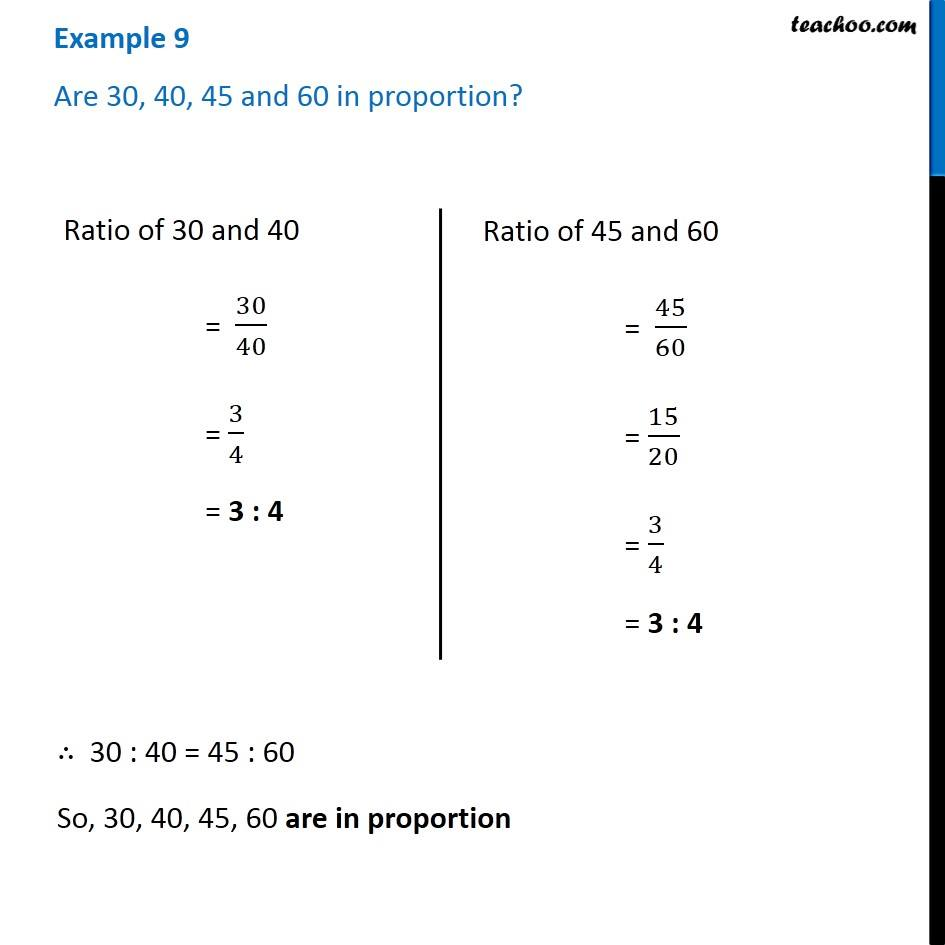Proportion

Chapter 12 Class 6 Ratio and Proportion
Concept wiseLearn in your speed, with individual attention - Teachoo Maths 1-on-1 Class

### Transcript

Example 9 Are 30, 40, 45 and 60 in proportion? Ratio of 30 and 40 = 30/40 = 3/4 = 3 : 4 Ratio of 45 and 60 = 45/60 = 15/20 = 3/4 = 3 : 4 Ratio of 45 and 60 = 45/60 = 15/20 = 3/4 = 3 : 4 ∴ 30 : 40 = 45 : 60 So, 30, 40, 45, 60 are in proportion